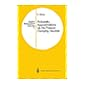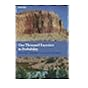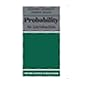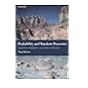Normal view MARC view ISBD view

# One thousand exercises in probabilityNo cover image available No cover image available519.2 ALD Probability approximations via the Poisson clumping heuristic 519.2 ELW From local times to global geometry, control and physics : Symposium on stochastic differential equations and applications : Papers 519.2 GOO The Estimation of probabilities. An essay on modern Bayesian methods. 519.2 GRI One thousand exercises in probability 519.2 GRI Probability : an introduction 519.2 GRI Probability and random processes: problems and solutions. 519.2 GRI Probability and random processes